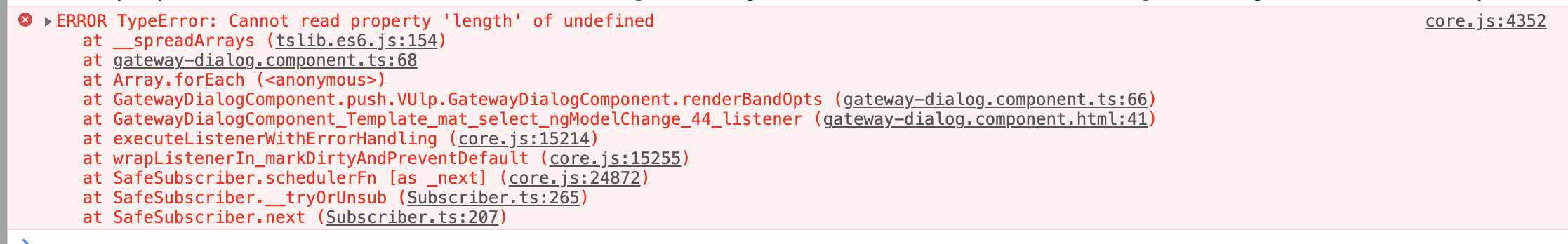21

2021

# ts的好处？举个实际例子

## 进入正题

Ts可以看作js的超集，主要相比于js，ts是一种强类型语言，针对变量等做了很多类型等的规范要求，规避了一些可能发生的错误

``````let frequency = (Number(start) + Number(interval) * index) / 1000000;
//@ts-ignore
frequency = _.isInteger(frequency) ? frequency : frequency.toFixed(1);
``````

``````let frequency = (Number(start) + Number(interval) * index) / 1000000;
frequency = _.isInteger(frequency) ? +frequency : +frequency.toFixed(1);
````````````this.refsdata.bands.forEach(b => {
// 通过type寻找相应的freqs
this.refsdata.bandList = [
//@ts-ignore
...this.bandList,
...this.onFindFreqs(b.freqs, entity.gtwtype)?.segments,
];
});
``````

``````this.refsdata.bands.forEach(b => {
// 通过type寻找相应的freqs
this.refsdata.bandList = [
...this.refsdata.bandList,
...this.onFindFreqs(b.freqs, entity.gtwtype)?.segments,
];
});
``````

1.少用//@ts-ignore
2.人类的本质就是真香

TAG